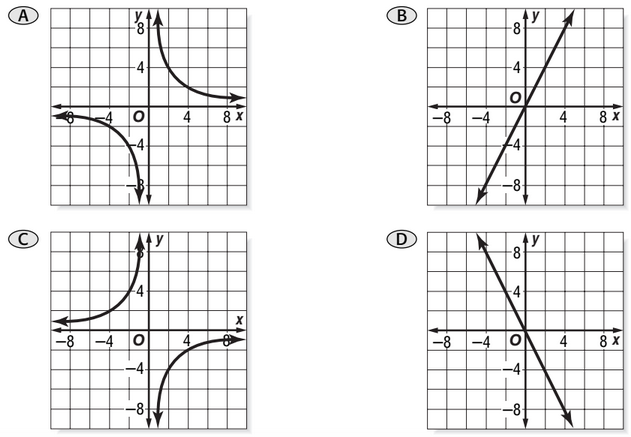### Day 64 - Rational Applications - 11.19.14

 UpdatesUnit 4 Test11/21Summative Exam 211/25Bell Ringery is directly proportional to x and y = 24 when x = 4. What is the value of y when x = 3?18232043none of the abovey is inversely proportional to x and y = 8 when x = 3. What is the value of y when x = 4?69710.67none of the aboveThe time (t days) required to build a house is inversely proportional to the number of builders (n), all working at the same rate. If there are 6 builders, it takes 80 days to complete the house. How many builders must be employed to build the house in just 16 days?1.2253036none of the aboveDetermine the constant of variation if y varies inversely as x and y = 4.25 when x = -1.3-3.269-5.525-0.306-2.950none of the aboveIdentify the graph of xy = k if x = -2 when y = -4.ReviewPrerequisitesDividing by 0Rational NumbersNumerator and DenominatorPolynomialsRational FunctionsPolynomial / PolynomialExcluded ValuesGraphs at Excluded ValuesSimplifying Rational ExpressionsMultiplying/Dividing Rational ExpressionsUnit Conversions (Dimensional Analysis)Dividing PolynomialsLong Division of PolynomialsAdding/Subtracting Rational ExpressionsMixed Expressions and Complex FractionsSolving Rational EquationsVariationDirectInverseLessonExit TicketPosted on board at end of block. Lesson Objective(s)How are direct and inverse variations related?How can direct and inverse variation problems be solved?Standard(s)Solving Rational Equations - 12.9CC.9-12.A.CED.1 Create equations that describe numbers or relationship. Create equations and inequalities in one variable and use them to solve problems. Include equations arising from linear and quadratic functions, and simple rational and exponential functions.CC.9-12.A.REI.11 Represent and solve equations and inequalities graphically. Explain why the x-coordinates of the points where the graphs of the equations y = f(x) and y = g(x) intersect are the solutions of the equation f(x) = g(x); find the solutions approximately, e.g., using technology to graph the functions, make tables of values, or find successive approximations. Include cases where f(x) and/or g(x) are linear, polynomial, rational, absolute value, exponential, and logarithmic functions.CC.9-12.A.REI.2 Understand solving equations as a process of reasoning and explain the reasoning. Solve simple rational and radical equations in one variable, and give examples showing how extraneous solutions may arise.Dividing Polynomials - 12.5CC.9-12.A.APR.6 Rewrite rational expressions. Rewrite simple rational expressions in different forms; write a(x)/b(x) in the form q(x) + r(x)/b(x), where a(x), b(x), q(x), and r(x) are polynomials with the degree of r(x) less than the degree of b(x), using inspection, long division, or, for the more complicated examples, a computer algebra system.Mathematical Practice(s)#1 - Make sense of problems and persevere in solving them#2 - Reason abstractly and quantitatively#4 - Model with mathematics#5 - Use appropriate tools strategically#7 - Look for and make use of structurePast CheckpointsRational ExpressionsCheckpointsSimplifying Rational ExpressionsCheckpointsMultiplying/Dividing Rational ExpressionsCheckpointsDividing PolynomialsCheckpointsAdding/Subtracting Rational ExpressionsCheckpointsMixed Expressions and Complex FractionsCheckpointsSolving Rational EquationsCheckpointsVariationCheckpoints